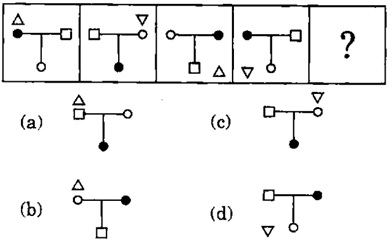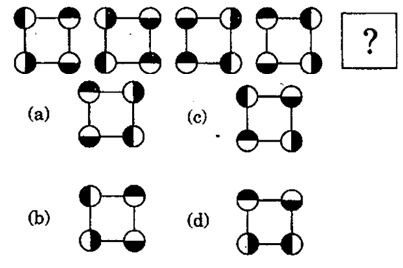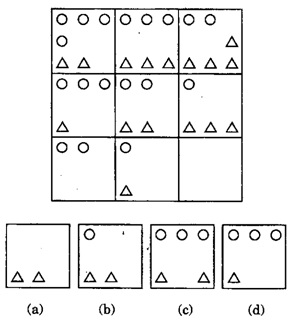# Non Verbal Reasoning Questions and Answers

## Non Verbal Reasoning Questions and Answers

Learn and practice the chapter "Non Verbal Reasoning" with these solved Logical Reasoning Questions and Answers. Each question in the topic is accompanied by a clear and easy explanation, diagrams, shortcuts and tricks that help in understanding the concept.

## Use of Non Verbal Reasoning Questions

The questions and examples given in this section will be useful to all the freshers, college students and engineering students preparing for placement tests or any competitive exam like MBA, CAT, MAT, SNAP, MHCET, XAT, NMAT, GATE, Bank exams - IBPS, SBI, RBI, RRB, SSB, SSC, UPSC etc.

Practice with this online test to ensure that you get through your placements and entrance tests with flying colours!
1. Out of the given answer figures, which is the correct one to replace the question mark?a. A
b. C
c. B
d. D

Explanation:

First a 6-sided figure encloses (6-1=)5-sided figure.
Then a 5-sided figure encloses (5-1=)4-sided figure.
So on,
The answer must be a 3-sided figure enclosing a 2-sided figure. So answer figure D should be next. So, answer is option D

2. Out of the given answer figures, which is the correct one to replace the question mark?a. B
b. A
c. C
d. D

Explanation:

The triangle shifts one place clockwise and also inverts every time it shifts. That gives options A and B.
The dark circle shifts anti-clockwise so now it will be below. So answer figure (a) is correct. So, Option b is correct answer.

3. Out of the given answer figures, which is the correct one to replace the question mark?a. A
b. D
c. B
d. C

Explanation:

The shaded and light parts of each circle inverse (interchange) to give us the 2nd diagram. Then both circles on right side simply change positions with left side circles without inversion of shaded parts. Now again the shaded and light parts of each circle inverse their positions. Again, circles on right should exchange positions with circles with left without inversion. So, answer figure (b) is correct. So, answer is option c

4. Out of the given answer figures, which is the correct one to replace the empty box?a. D
b. C
c. B
d. A